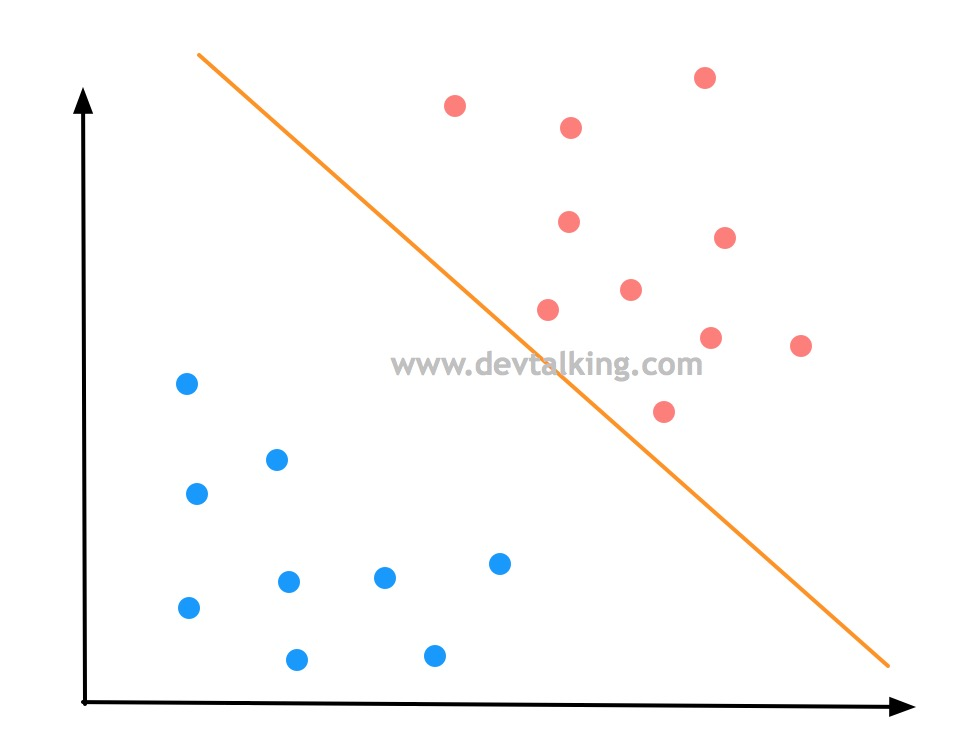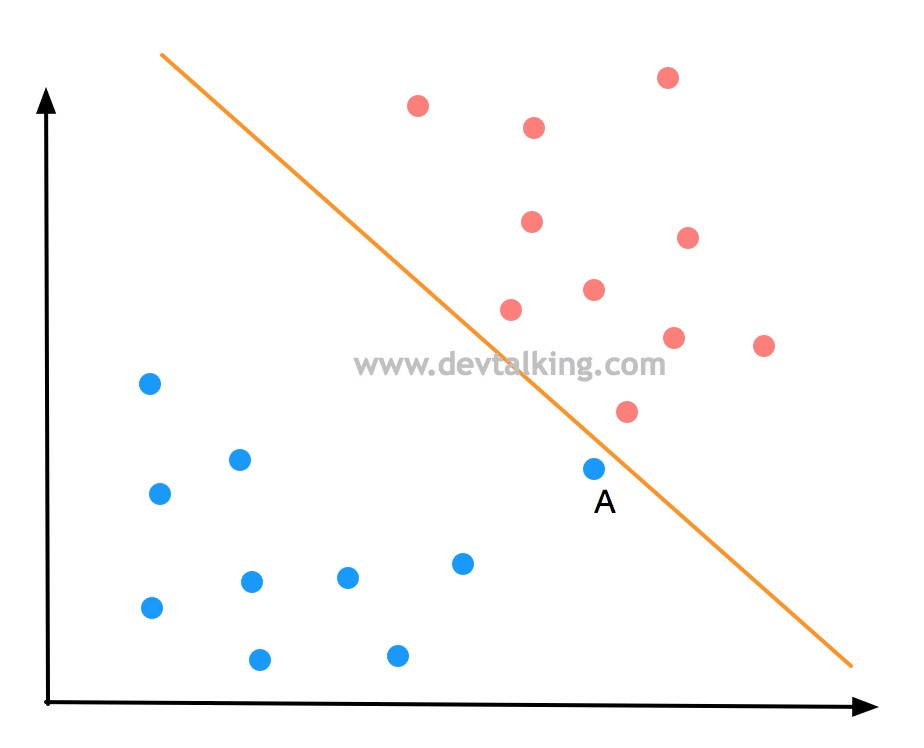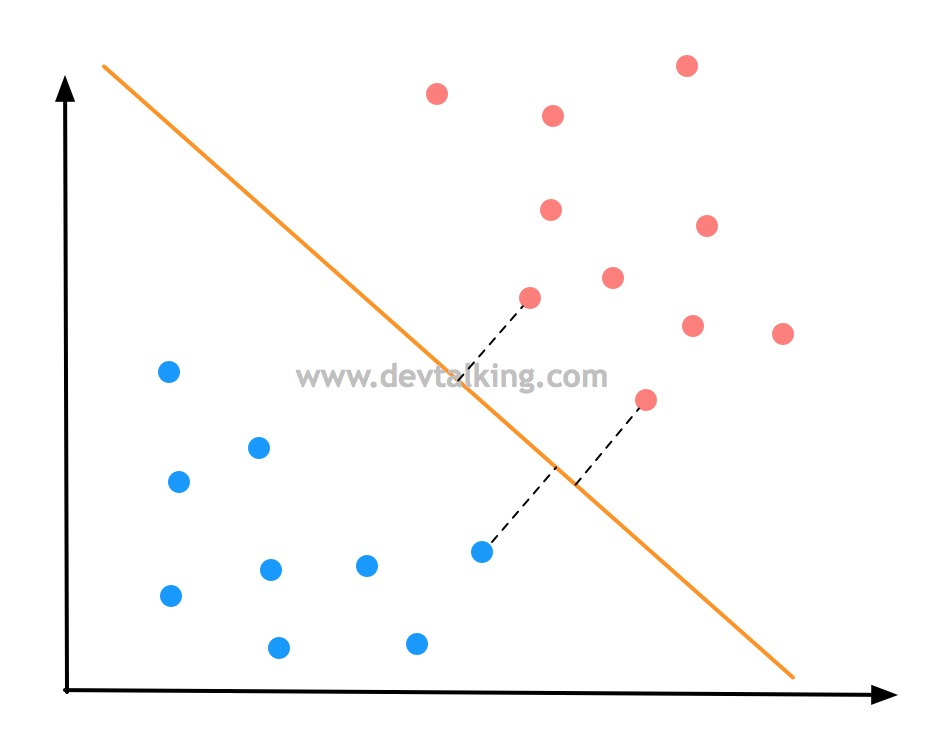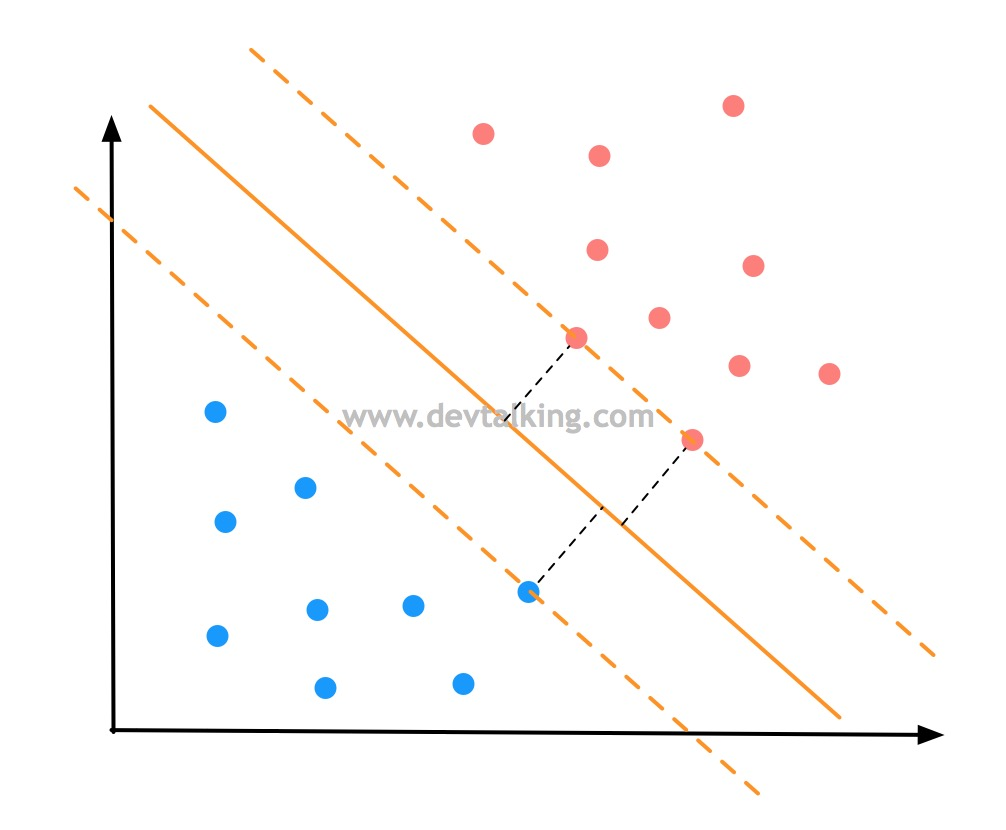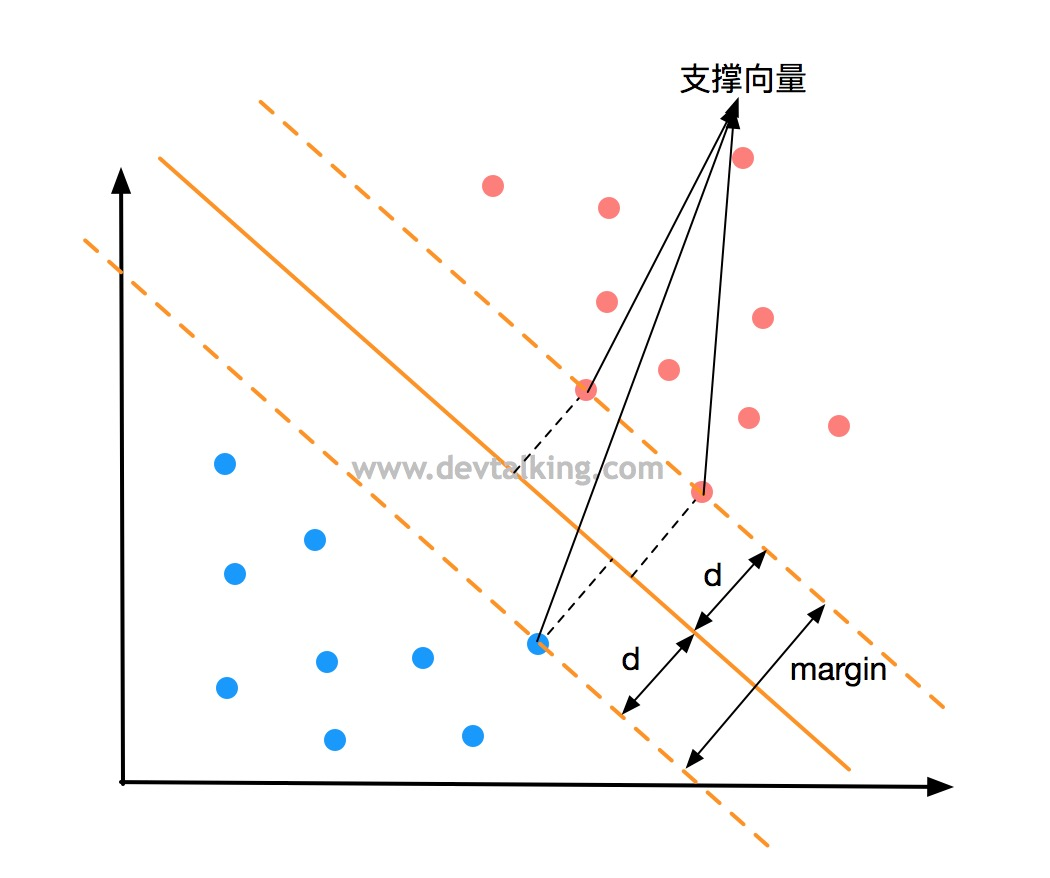## 基尼系数

$$G = 1 - \sum_{i=1}^k p_i^2$$

$$\{\frac 1 3,\frac 1 3,\frac 1 3\}$$

$$G = 1-(\frac 1 3)^2-(\frac 1 3)^2-(\frac 1 3)^2=0.6666$$

$$\{\frac 1 {10},\frac 2 {10},\frac 7 {10}\}$$

$$G = 1-(\frac 1 {10})^2-(\frac 2 {10})^2-(\frac 7 {10})^2=0.46$$

## 什么是决策树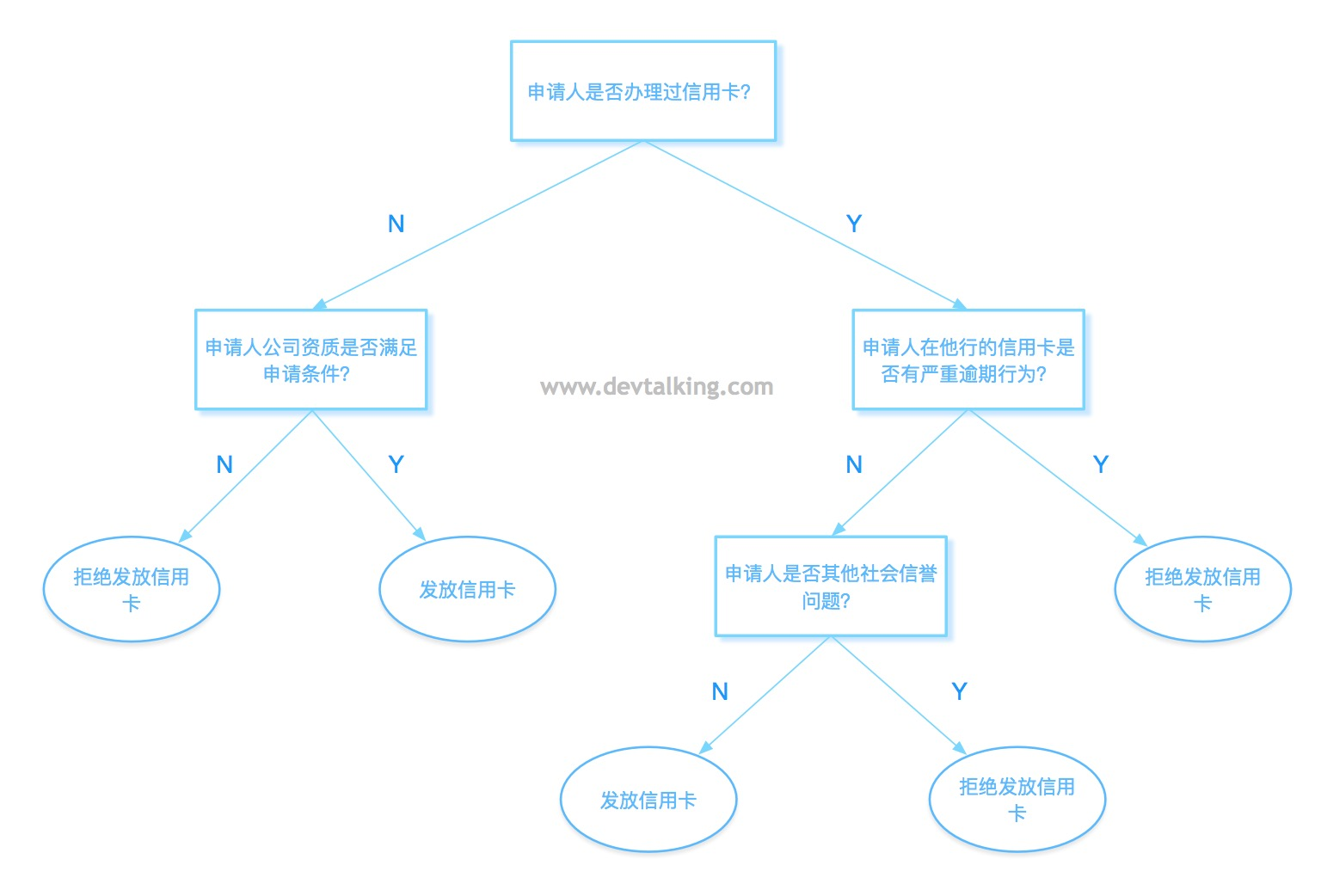## SVM处理非线性问题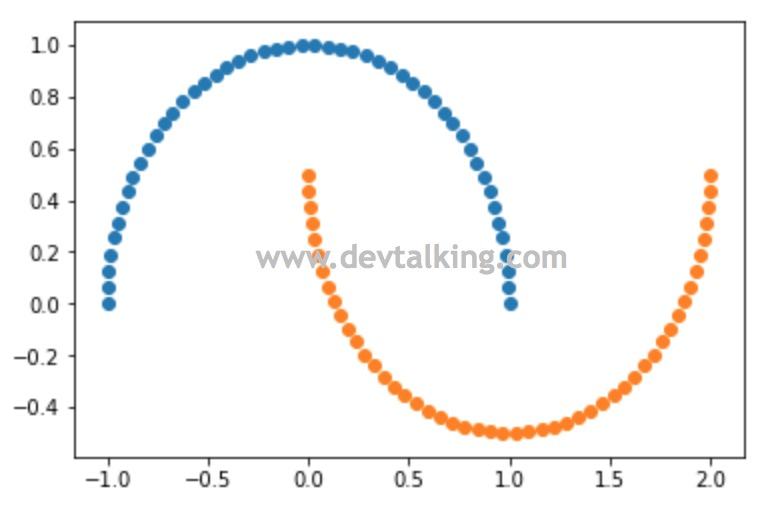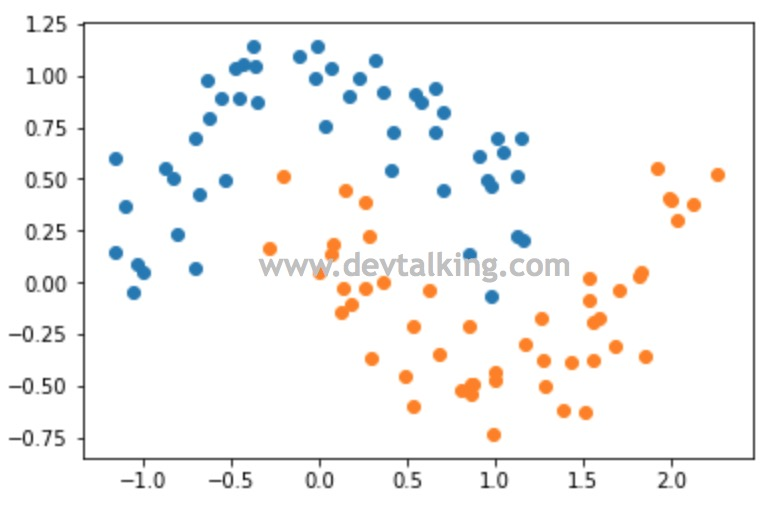• 多项式自然要用到之前讲过的PolynomialFeatures类。
• 数据归一化需要用到StandardScaler类。
• 同样需要用到Pipeline将多个过程封装在一个函数里，所以要用到Pipeline类。

## 什么是SVM